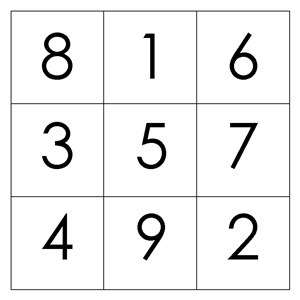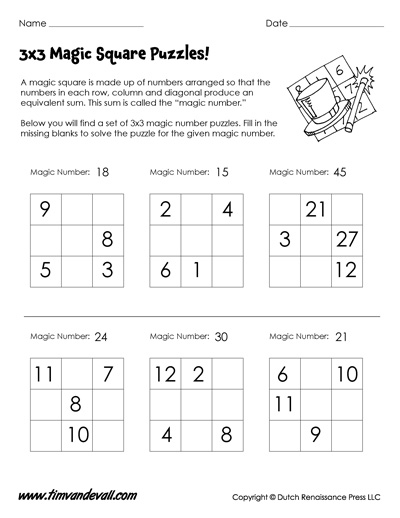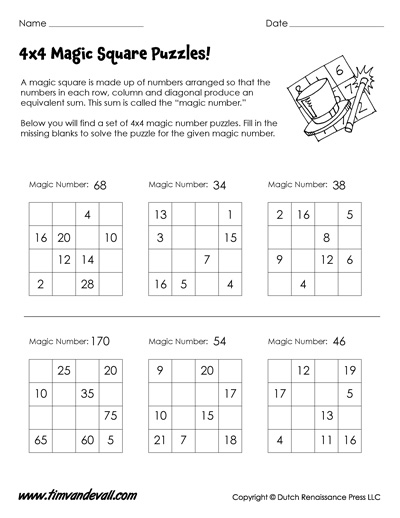# Magic Squares Worksheets | 3×3 & 4×4 Magic Square Puzzles

A magic square is a square grid containing numbers, arranged so that the numbers in each row, column and diagonal produce an equivalent sum. This sum is called the “magic number.” A magic square has the same number of rows and columns, and it is possible to create a magic square of all sizes except 2×2. The simplest unique magic square is made with a 3×3 grid.

Here is an example of a 3×3 magic square, where the magic number is 15:## Printable Magic Squares Worksheets

If you are a math student or teacher interested in magic squares, please take a look at the magic square worksheets below. Each worksheet is available as a free printable in jpeg format. Click a worksheet image to visit its corresponding download page. A link to the answer key is also included there.

## 3×3 Magic Square Worksheet## 4×4 Magic Square WorksheetIf you enjoyed solving these magic squares, why not try your hand at making a few of your own? It’s a fun challenge, but achievable with a bit of effort, especially when the grid size is relatively small.

For more information on magic squares, and additional relevant content, please see:

Magic Squares – Wikipedia: https://en.wikipedia.org/wiki/Magic_square

Save